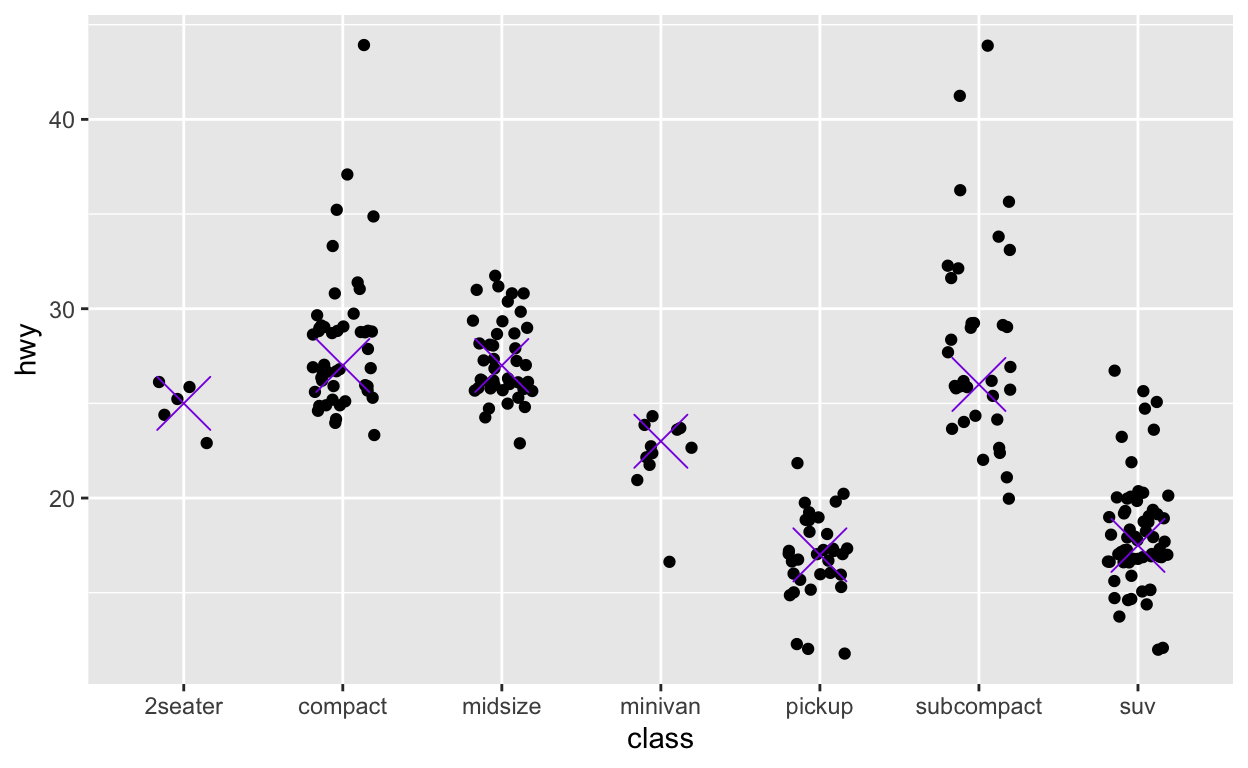# 6: Exploratory Analysis

Data visualization, part 1. Code for Quiz 7.

1. Load the R package we will use
``````library(tidyverse)
``````

# Question: modify slide 34

``````ggplot(faithful) +
geom_point(aes(x = eruptions, y = waiting, colour = waiting < 64))
``````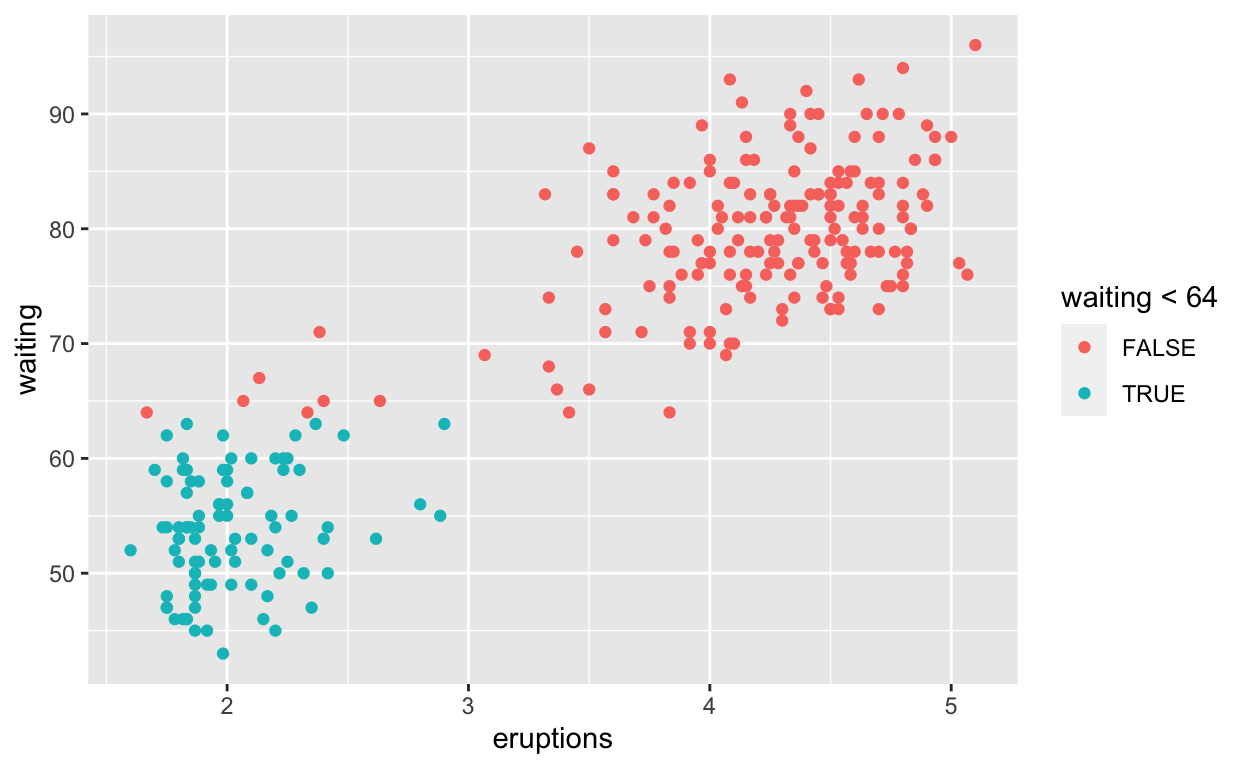``````ggsave(filename = "preview.png", path = here::here("_posts", "2021-03-30-exploratory-analysis"))
``````

# Question: modify intro-slide 35

``````ggplot(faithful) +
geom_point(aes(x = eruptions, y = waiting),
colour = 'dodgerblue')
``````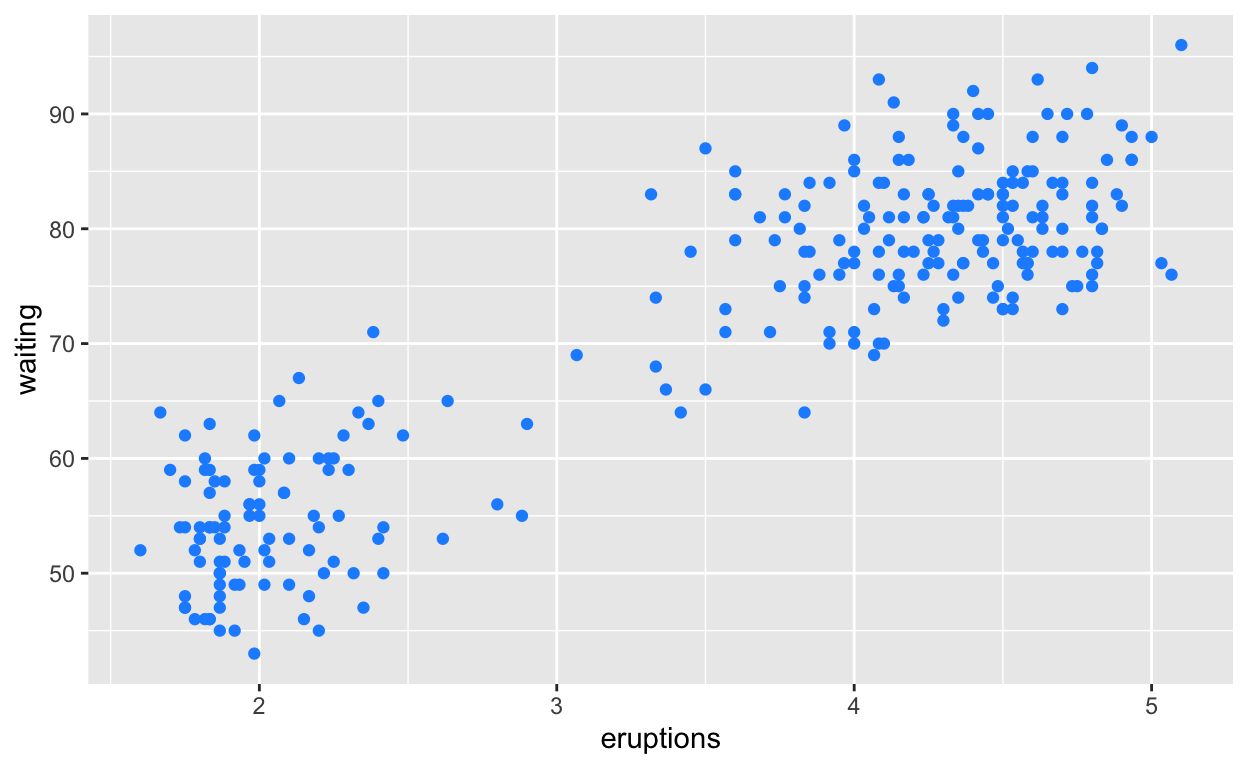# Question: modify intro-slide 36

``````ggplot(faithful) +
geom_histogram(aes(x = waiting))
``````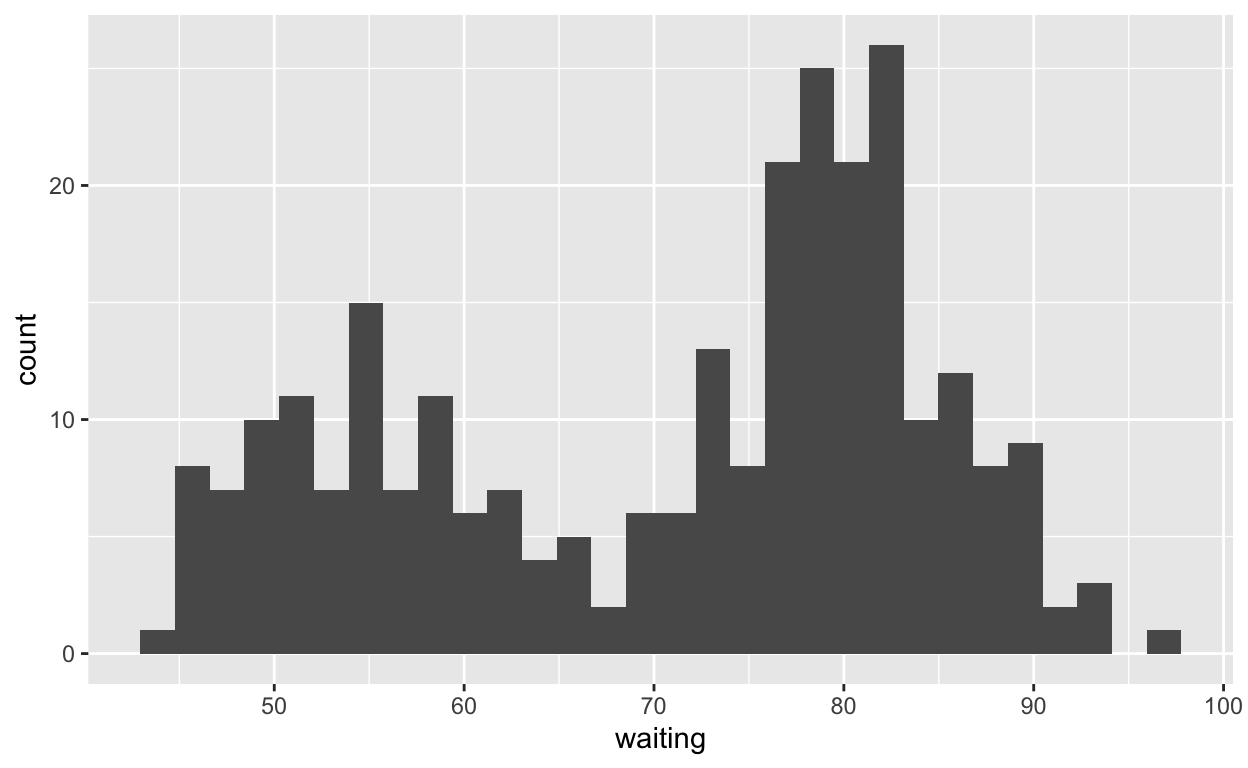# Question: modify geom-ex-1

``````ggplot(faithful) +
geom_point(aes(x = eruptions, y = waiting),
shape = "asterisk", size = 8, alpha = 0.7)
``````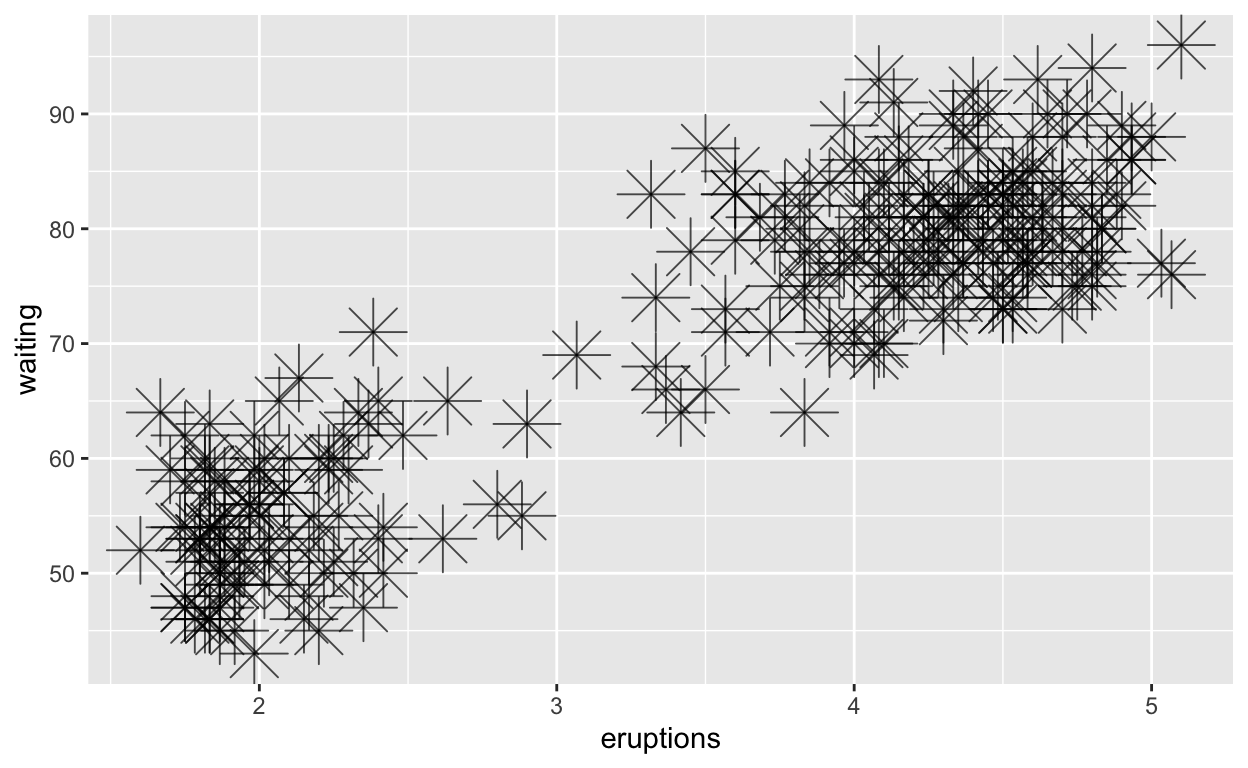# Question: modify geom-ex-2

``````ggplot(faithful) +
geom_histogram(aes(x = eruptions, fill = eruptions > 3.2))
``````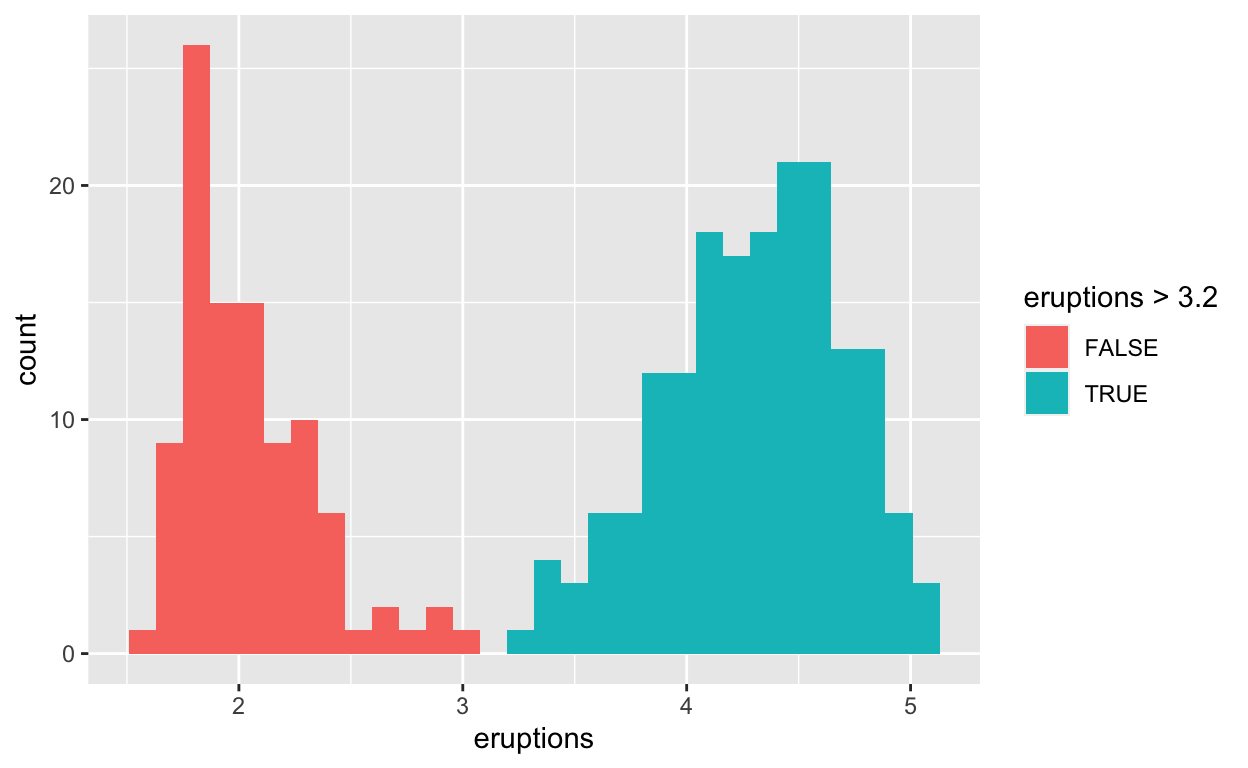# Question: modify stat-slide-40

``````data("mpg")

# variable definitions
# ?mpg

# mpg  %>% glimpse()

ggplot(mpg) +
geom_bar(aes(x = manufacturer))
``````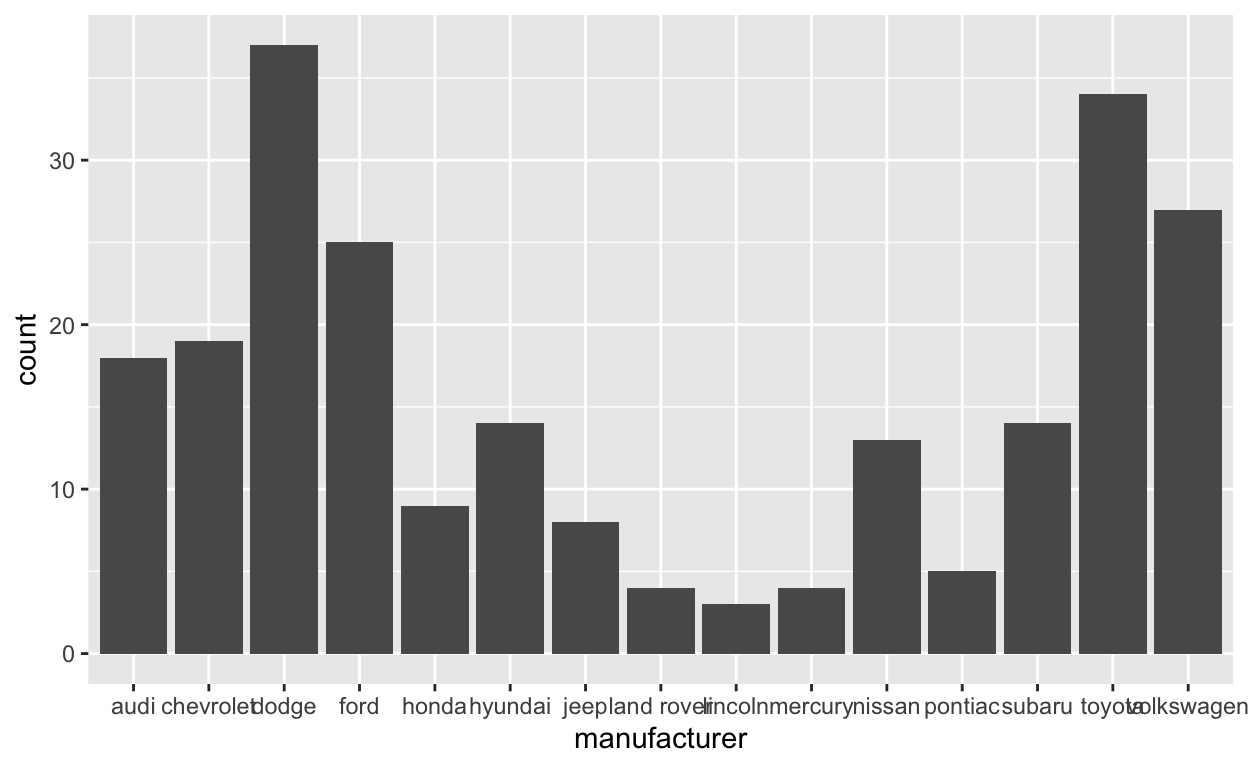# Question: modify stat-slide-41

``````mpg_counted <- mpg %>%
count(manufacturer, name = 'count')
ggplot(mpg_counted) +
geom_bar(aes(x = manufacturer, y = count), stat = 'identity')
``````# Question: modify stat-slide-43

``````ggplot(mpg) +
geom_bar(aes(x = manufacturer, y = after_stat(100 * count / sum(count))))
``````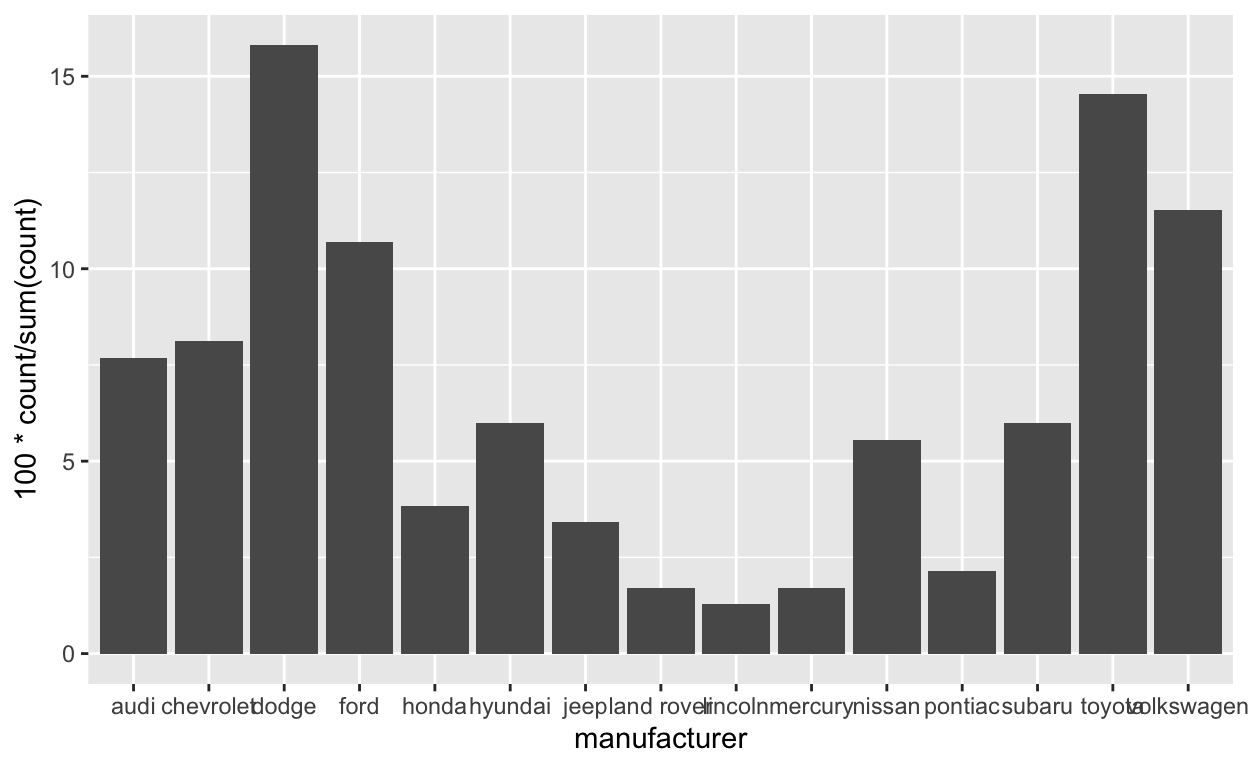# Question: modify answer to stat-ex-2

``````ggplot(mpg) +
geom_jitter(aes(x = class, y = hwy), width = 0.2) +
stat_summary(aes(x = class, y = hwy), geom = "point",
fun = "median", color = "blueviolet",
shape = "cross", size = 9 )
``````